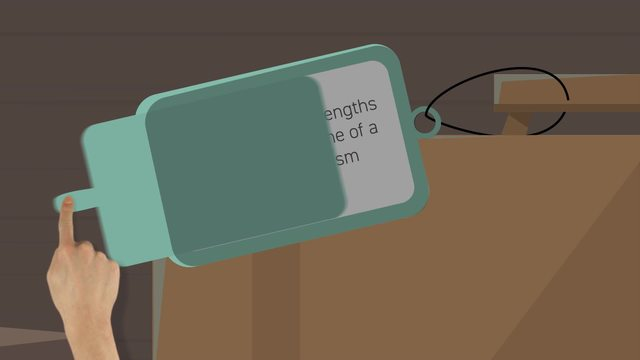# How Scaling Side Lengths Changes the Volume of Rectangular PrismsRating

Ø 5.0 / 1 ratings
The authorsChris S.

## Basics on the topicHow Scaling Side Lengths Changes the Volume of Rectangular Prisms

After this lesson you will be able to multiply or divide the dimensions of a rectangular prism to reduce or enlarge its volume.

The lesson begins with calculating the volume of a rectangular prism. It leads to enlarging the prism by multiplying the original volume by two cubed. It concludes with reducing the prism by multiplying the original volume by one third cubed.

Learn how to change the volume of a rectangular prism by helping Morton adjust his luggage size for his flight to Germany!

This video includes key concepts, notation, and vocabulary such as: scale factor, k (the amount by which the dimensions are multiplied); enlargement (when k is greater than 1); reduction (when k is less than 1); and the formula for the volume of a prism that is being scaled by a factor of k (volume equals k cubed times the original volume).

Before watching this video, you should already be familiar with calculating the volume of a rectangular prism using the formula, volume equals length times width times height, or volume equals base area times height.

After watching this video, you will be prepared to solve composite volume problems.

Common Core Standard(s) in focus: 6.G.A.2 A video intended for math students in the 6th grade Recommended for students who are 11-12 years old

### TranscriptHow Scaling Side Lengths Changes the Volume of Rectangular Prisms

Morton is an up and coming stamp collector who's making an impression. He's been invited to present his stamps at a conference in Berlin, Germany! He's going to fly overseas for the very first time. Morton is shocked by the high baggage check fees. But Morton has a clever way to avoid the high costs. His luggage can adjust in size! In order to make sure his luggage is just the right size Morton will need to scale the side lengths to change the volume of a rectangular prism. To begin with, Morton's bag is 24 inches long, 12 inches wide, and 15 inches tall. What's the volume of Morton's bag? The volume is length times width times height. That's 24 times 12 times 15. That might not be enough! Morton is considering bringing his entire stamp collection to Berlin. In that case, he can enlarge his bag by doubling the dimensions. How would that affect the volume? The dimensions of the enlarged bag are 2 times 24, by 2 times 12, by 2 times 15. The volume is the product of these new dimensions. Multiplying everything together, the result is 34,560 inches cubed! Even though we only doubled the dimensions, that's a lot bigger than the volume of the original! What happened? Let's look again at our calculation of the enlarged volume. We multiplied each dimension of the original bag by 2. Let's group those together and multiply them to get 2 cubed. So the volume of the enlarged bag is 2 cubed times the original volume. So the volume of the enlarged bag is 2 cubed times V. If we multiply each dimensions of the prism by 2, the volume increases by a factor of 2 cubed. And that's why the volume of the enlarged bag is so much bigger than the original. Morton can fit his entire stamp collection in the enlarged bag, but the plane will charge him an arm and a leg! Morton stamps out that idea. Instead, he'll reduce the bag's dimensions, and take only the most valuable stamps with him. Remember that the dimensions of the original are 24 by 12 by 15. Morton wants to reduce the bag by one-third. That means each dimension gets multiplied by one-third. What's the volume of the reduced bag? It's one-third times 24 by one-third times 12 by one-third times 15. A third of 24 is 8, a third of 12 is 4, and a third of 15 is 5. So the volume of the reduced bag is only 160 cubic inches! Why so tiny? Let's look again at our calculation of the reduced volume. Each dimension was multiplied by one-third. Grouping them as before shows us that the volume of the reduced bag is one-third cubed times the volume of the original. So the volume of the reduced bag is one-third cubed times V. This tiny bag gets Morton's stamp of approval! Let's review how scaling the dimensions of a prism affected the volume. When we scaled the dimensions by 2 the volume was 2 cubed times the original. When we scaled the dimensions by one-third the new volume was one-third cubed times the original. In general, if each dimension is scaled by a factor of 'k', the new volume is 'k' cubed times the original volume. The scale factor can be any real number greater than 0. Morton and his precious cargo arrive in Berlin! Morton waits anxiously to collect his tiny bag of rare stamps. Oh no! I guess the customs' officials want to stamp their impression on Morton's collection!